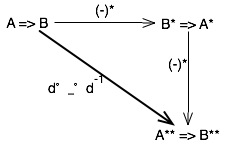# The Categorical Model of Linear Logic

Today we’ll finally get to building the categories that provide the model for
the multiplicative linear logic. Before we jump into that, I want to explain why it is that we separate out the multiplicative part.
Remember from the simply typed lambda calculus, that [we showed that the type system was precisely a subset of intuitionistic logic][lambda-type], and that programs in the lambda calculus corresponded to proofs in the type system. In particular, it was propositional intuitionistic logic using only the “→” operation. Similarly, if you build up a more interesting typed lambda calculus like [System-F][systemf], the type system is intuitionist logic *with* the “∀” quantifer and the “∧” operator, but without “∨”, negation, or “∃”. Why do we eliminate the existentials and friends? Because if we left them in, then the *type system* becomes Turing complete and undecidable. If we’re careful, we can analyze a program using universal types, logical and (composition in lambda), and implication and infer the types if it’s a valid program. (Actually, even System-F is undecidable without any type annotations, but HMF – the Hindley-Milner subset, *is* decidable. NP-hard, but decidable.)
The main reason that people like me really care about linear logic is because there is a variant of lambda calculus that includes *linear types*. Linear types are types where referencing the value corresponding to the type *consumes* it. For dealing with the semantics of real programming languages, there are many things that make sense as linear types. For example, the values of an input stream behave in a linear way: read something from an input stream, and it’s not there anymore: unless you copied it, to put it someplace safe, it’s gone.
For typing linear lambda calculus, we use *intuitionistic linear logic* with the *multiplicative* operators. They give us a *decidable* type system with the capability to express linear type constraints.
Enough side-tracking; back to the categories.
We can now finally define a *linear category*. A category C is a linear category if it is both a [cartesian category][cartesian] and a [monoidal][monoidal] [closed category][monclose] with a *dualizing functor*. A dualizing functor is a *contravariant* closed functor defining properties of the categorical exponential, written (-)* : C → C. (That should be read with “-” as a placeholder for an object; and * as a placeholder for an exponent.) (-)* has the property that there is a natural isomorphism d : Id ≡ (-)** (That is, d is an identity natural isomorphism, and it’s equivalent to applying (-)* to the result of applying (-)* ) such that the following commutes:So, what does this mean? Take the linear implication operator; mix it with the categorical exponent; and what you get *still* behaves *linearly*: that is, if “X -o Y”; that is, one X can be consumed to produce one Y, then 2 Xs can be consumed to produce 2 Ys; and N Xs can be consumed to produce N Ys.
So a linear category behaves cleanly with exponents; t has a linear implication; it has an *eval* operator (from the fact that it’s a cartesian category) to perform the linear implications; it has tensor for producing groups of resources. That’s it; that’s everything we need from categories for a model of linear logic.
Now, there’s just one more question: are there any real linear categories that make sense as a model for linear logic? And obviously, the answer is yes. There are two of them, called **CPO** and **Lin**.
**CPO** is the category with continuous partial orders as objects, and monotonic functions as morphisms. Think about that, and it makes a whole lot of sense for linear logic; the equivalence classes of the partial order are resources of the same “value” (that is, that can be exchanged for one another); and you can’t climb *upwards* in the partial order without adding something.
The other one, **Lin**, is a lot more complicated. I’m not going to explain it in detail; that would lead into another two-month-long series! But to put it briefly, **Lin** is the category with *coherent domains* as objects, and linear maps as morphisms. To make that make sense, I would have to explain domain theory, which (to put it mildly) is complicated; the short version is that a domain is a kind of CPO. But the reason we care about **Lin** is that programming language semantics are generally described using [*denotational semantics*][denote]; and denotational semantics are built using a kind of domain, called a Scott domain. So this gives us a domain that we can use in programming language semantics with exactly the properties we need to explain how linear types work.
[lambda-type]: http://goodmath.blogspot.com/2006/06/finally-modeling-lambda-calculus.html
[systemf]: http://en.wikipedia.org/wiki/System_F
[cartesian]: http://scienceblogs.com/goodmath/2006/06/categories_products_exponentia_1.php
[monclose]: http://scienceblogs.com/goodmath/2006/07/towards_a_model_for_linear_log_1.php
[monoidal]: http://scienceblogs.com/goodmath/2006/07/the_category_structure_for_lin_1.php
[denote]: http://en.wikipedia.org/wiki/Denotational_semantics